Maths-
General
Easy

Question

# Sides of a triangle are in the ratio 12 : 17 : 25 and its perimeter is 540 cm. Find its area.Hint:

## The correct answer is: 9000 cm2

### It is given that Perimeter of triangle = 540 cm⇒ 12x + 17x + 25x = 540⇒  54x = 540⇒  x = 10So, a = 120 cm , b = 170 cm , c = 250 cmUsing Heron’s formula,Area of triangle =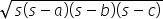where s =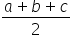s =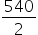= 270Area of triangle=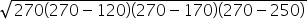=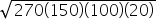=  9000 cm2#### With Turito Foundation.#### Get an Expert Advice From Turito.